Create a new printableGeometry
Math Worksheets

Sample - Click above to make a new math worksheet (PDF).
 Name _____________________________Date ___________________
Plane Figures
Name each figure using letters and symbols.
 1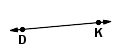2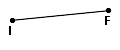3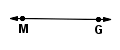Draw and label an example of each.
4.SL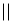DI
5.
 line WI
6.
 line segment HK

Classify each pair of lines as parallel, intersecting, or perpendicular.
7.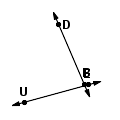8.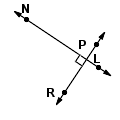9.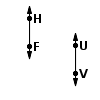10.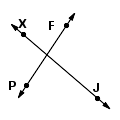Classify each angle as acute, obtuse, straight, or right.
 11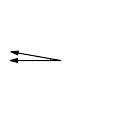12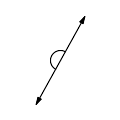13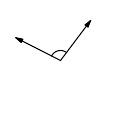14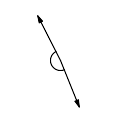Key #2
 aKey #2
Write polygon or not a polygon. For a polygon, name the type of polygon and also write regular or not regular.
 15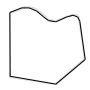16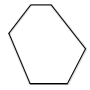17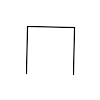18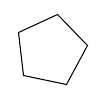Classify each triangle as isosceles, scalene, or equilateral and as right, acute, or obtuse.
19.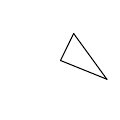20.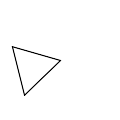21.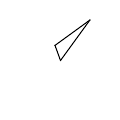22.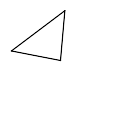Classify each triangle as isosceles, scalene, or equilateral by the lengths of its sides.
23.
 3 cm, 18 mm, 7 cm24.
 16 cm, 9 cm, 9 cm25.
 10 in, 24 in, 24 inName the part of the circle. Write chord, radius,center, or diameter.
26.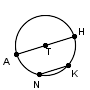NK27.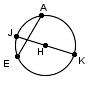JK28.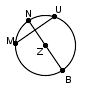UM29.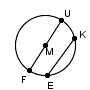FM